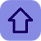## Basics of Sub-netting

Posted by Tushar Bedekar

### What is Subnetting?

To subnet a network is to create logical divisions of the network. Subnetting, therefore, involves dividing the network into smaller portions called subnets. Subnetting applies to IP addresses because this is done by borrowing bits from the host portion of the IP address. In a sense, the IP address then has three components - the network part, the subnet part and, finally, the host part.
For Example:
11111111.11111111.11111111.11110000 will be equal to 255.255.255.240 in decimal.
And, 11110000 = 240
In 11111111 the total bits in network field are 8. So, 2^8=255   and,

For 11110000, Notice that the fourth byte in the network mask has four bits set to ‘1’. If you look at the chart below, its value is 240 (128+64+32+16).
There are so many reasons why we subnet:
a. It helps in the preservation of address space in other not to waste addresses.
b. It used for security.

c. It helps to control network traffic due to collisions of packets transmitted by other node (host) on the same segment.

In order to subnet a network address, The  subnet mask has to be extended, using some of the bits from the host ID portion of the address to create a sub network ID.

### How to Subnet:

This mean, borrow some bits from the host part to add to the network part. This allows us to have more networks than using the default subnet mask. For example, we can borrow some bits in the next octet to make the address belong to a different network.
For example:
Now you can clearly see that the subnet mask will decide which is the network part, which is the Host part. By borrowing 8 bits, our subnet mask will be like this:
After changing the second octet of the subnet mask from all “0″ to all “1″, the Network part is now extended. Now we can create new networks by changing number in the first or second octet. This greatly increases the number of networks we can create.
So, in conclusion we “subnet” by borrowing bit “0″ in the Host portion and converting them to bit “1″. The number of borrowed bits is depended on how many networks we need.
For Example:
Calculating Host: 11111111.11111111.11111111.11110000 will be equal 14 hosts
Notice the last four bits of the network mass has set to 0,
Formula = 2^4=16, and out of 16 we have only 14 valid hosts.
16-2=14 hosts, we have subtracted 2 because it is used for broadcast and network ID in the network.
Below is a quick reminder of the two major rules governing the number of subnet valid host addresses available given the particular network mas length.
1. Number of Available Subnet Cheat Sheet
Where “S” = Number of Subnet bits
2. Number of Available Valid Host Addresses Cheat Sheet
Where “h” = Number of host bits
3. Binary/Decimal/Subnet Cheat Sheet.